# Test: Chemical Kinetics - 2

## 25 Questions MCQ Test Topic-wise MCQ Tests for NEET | Test: Chemical Kinetics - 2

Description
Attempt Test: Chemical Kinetics - 2 | 25 questions in 35 minutes | Mock test for NEET preparation | Free important questions MCQ to study Topic-wise MCQ Tests for NEET for NEET Exam | Download free PDF with solutions
QUESTION: 1

### Study of rate of chemical reaction is known as

Solution:

Chemical Kinetics deals with rate of a Chemical reactions.

QUESTION: 2

### Which among the following is an instantaneous reaction?

Solution:

Precipitation of AgCl is an example of instantaneous reaction.

QUESTION: 3

### The rate of reaction can be measured as

Solution:

Rate of reaction can be measured in terms of Rate of disappearance of reactant or rate of apperance of product.

QUESTION: 4

Rate of reaction for the combustion of propane is equal to

C3H8(g) + 5O2(g) → 3CO2(g) + 4H2O(g)

Solution: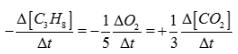QUESTION: 5

What is the order of a reaction which has a rate expression ; Rate =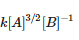Solution:

Total order of reaction = order wrt to A + order wrt to B.

QUESTION: 6

Molecularity of a reaction

Solution:

Molecularity is defined as the number of molecules, atoms, or radicals that must collide simultaneously in order for the reaction to take place. It is always a whole number (except zero) and cannot be negative.

QUESTION: 7

For which of the following reaction the units of rate constant and rate of the reaction are same?

Solution: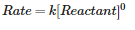QUESTION: 8

The rate of chemical reaction becomes double for every 10o rise in temperature because of

Solution:

With increase in temperature molecular collisions increases therefore rate of reaction doubles.

QUESTION: 9

The rate is independent of the concentration of the reactants in

Solution: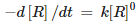QUESTION: 10

A reaction whose order is different from the actual due to large excess concentration of one of the reactants is called?

Solution:

A reaction whose order is different from the actual due to large excess concentration of one of the reactants is called pseudo 1st order reactions.

QUESTION: 11

The role of catalyst in a chemical reaction is to change the

Solution:

Role of catalyst is to decrease the activation energy of reaction.

QUESTION: 12

Rate of ionic reactions are generally

Solution:

Ionic reactions occur very fast.

QUESTION: 13

The metabolism of hormones in human body is an example of

Solution:

It is pseudo first order reaction.

QUESTION: 14

If a reaction proceeds with a uniform rate throughout, the reaction is

Solution:

Rate of reaction is independent of concentration of reactant.

QUESTION: 15

Which among the following is an example of photochemistry used in our daily life?

Solution:

Photography is an example of photochemistry used in our daily life.

QUESTION: 16

The ionic reactions are generally very fast because

Solution:

Ionic compounds in solution react faster than molecular compounds. This is because Ionic compounds break apart to form free ions. Therefore, there are no bonds to break so the reaction is fast. Covalent compounds, on the other hand, have to break bonds first as they react, so the reaction is often slower.

QUESTION: 17

The rate constant of the reaction at temperature 200 K is 10 times less than the rate constant at 400 K. The activation energy of the reaction is

Solution: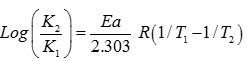QUESTION: 18

Which among the following is an instantaneous reaction?

Solution:

Precipitation of AgCl is an example of instantaneous reaction.

QUESTION: 19

The half life of a reaction is halved as the initial concentration of the reactant is doubled. The order of the reaction is

Solution:

For second order reaction half life is inversely related to concentration of reactant.

QUESTION: 20

If 75% of a first order reaction was completed in 32 min, then 50% of the reaction was completed in

Solution:

75% completion means 2 half lifes so 50% completion means only one half life.

QUESTION: 21

Milk turns sour at 40°C three times faster than it does at 0°C, this shows that activation energy of souring of milk (in cal) is

Solution: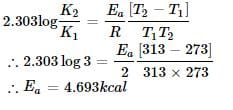QUESTION: 22

In a reaction, → Product, rate is doubled when the concentration of is doubled, and rate increases by a factor of 8 when the concentrations of both the reactants (Aand B) are doubled, rate law for the reaction can be written as

Solution:

The correct answer is option B
When conc. of B is doubled, the rate is doubled.
So, Order w.r.t.  [B] is 1.
The rate increases by a factor of 8 when conc. of [A] and [B] are doubled.
So, Order w.r.t.  [A] is 2.
Overall Rate is
Rate=k[A]2[B]

QUESTION: 23

Thermal decomposition of a compound is of first order. If 50% of a sample of a compound is decomposed in 120 min, the time taken for 90% completion is

Solution: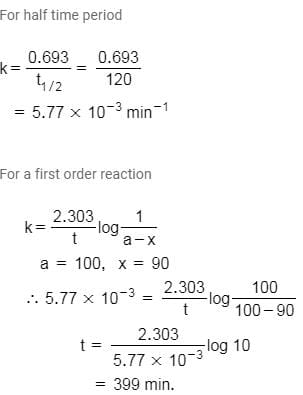QUESTION: 24

In the catalyzed decomposition of benzene diazonium chloride,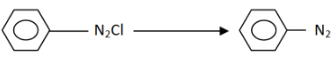Half life period is found to be independent of the initial concentration of the reactant. After 10 min, the volume of Ngas collected is 10 L and after the reaction is complete, it is 50 L. Hence, the rate constant of the reaction(in min-1) is

Solution: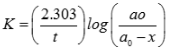QUESTION: 25

Decomposition of  H2Owas studied by titration against KMnOsolution. It was found that 0.4 mol of H2Owas reduced to 0.2 mol in 20 min and to 0.1 mol in 40 min and to 0.05 mol after 1 hr, the order of reaction must be

Solution:

For 1st order reaction,
Co  (t1/2)→Co/2  (t1/2)→Co/4  (t1/2)→…….Co/2n
Here, t1/2 = 20
So after 1st half life, concentration should be 0.4/2 = 0.2
After 2nd half life, concentration should be 0.4/4 = 0.1
This is correct with given data. So the order is 1st order.Use Code STAYHOME200 and get INR 200 additional OFF Use Coupon Code